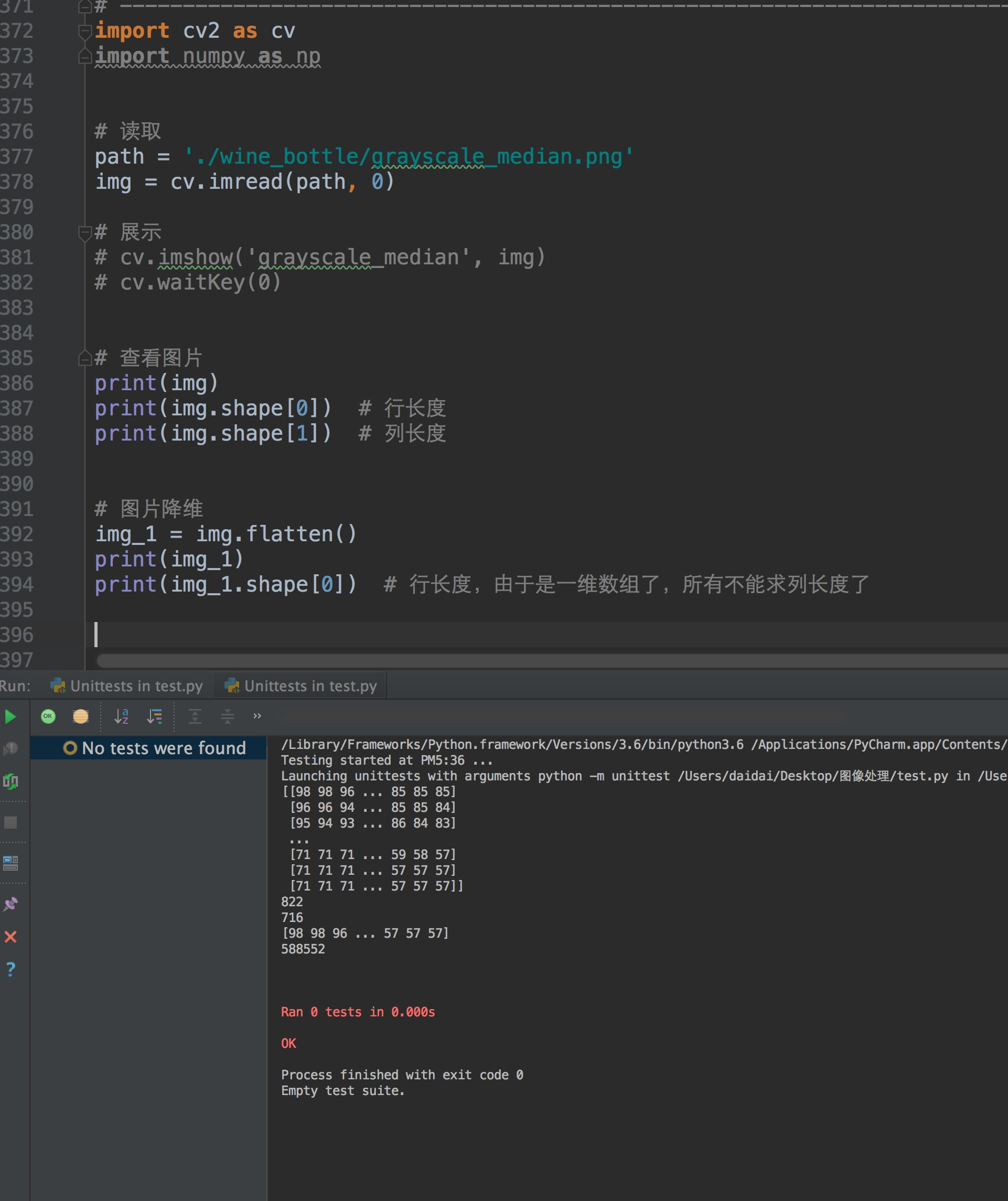• 二维数组降维处理 #假设是二维数组，进行降维处理 ins_data_list_2 = [] for y in ins_data_list: ins_data_list_2.append(str(y).replace('[','').replace(']','')) 保存一下简单模板 import json import requests ...
二维数组降维处理
     #假设是二维数组，进行降维处理
ins_data_list_2 = []
for y in ins_data_list:
ins_data_list_2.append(str(y).replace('[','').replace(']',''))

保存一下简单模板
import json
import requests
#from datetime import date
import time
import datetime
import pandas as pd
#import os
# 获取tenant_access_token
url = "https://open.feishu.cn/open-apis/auth/v3/tenant_access_token/internal/"
proxies = {
"https": "*****.com:8080"
}
post_data = {"app_id": "****","app_secret": "*****"}
r = requests.post(url, data=post_data, proxies=proxies)
tat = r.json()["tenant_access_token"]
#print(tat)

# 获取用户维度的用户活跃和功能使用数据
yesterday = str(datetime.date.today() + datetime.timedelta(days=-1))
df01 = pd.DataFrame()
pg_token = 0
have_more = True
header = {"content-type": "application/json", "Authorization": "Bearer " + str(tat), 'Connection': 'keep-alive', 'Referer':'https://open.feishu.cn/open-apis/admin/v1/'}
while have_more:
#  print(r.json())
pg_token = int(pg_token)+20
print(r.json())
reuser = r.json()["data"]
print(pg_token)
#  pg_token = reuser["page_token"]
have_more = reuser["has_more"]
print(have_more)
#  print(pg_token)
fsdt = r.json()["data"]["items"]
df = pd.DataFrame(reuser["items"])
df03 = df01.append(df)
df01 = df03
#  print(df01)
#print(df01)
df01.to_csv('D:/飞书接口/fs_user_data'+str(time.strftime('%Y%m%d', time.localtime()))+'.csv', index=0, sep='|')
}

展开全文python
• // // main.cpp // 二维数组 // 1.一个二维数组就像是若干个相同的数组放在一起，这对于存储多组...处理二维数组的时候直接当作一维数组就可以了每一行都是一个一维数组。 // 5.二维数组在存储的时候，是按照一行...

//
//  main.cpp
//  二维数组
//  1.一个二维数组就像是若干个相同的数组放在一起，这对于存储多组数据非常有用。
//  2.一维数组(One-Dimension Array)
//  3.二维数组(Two-Dimension Arrays)
//  4.处理二维数组的时候直接当作一维数组就可以了每一行都是一个一维数组。
//  5.二维数组在存储的时候，是按照一行接着一行来存储的，并不是在定义的时候，眼睛看到的那样的小方块，因此
//  二维数组是可以省略行号的，因为本来就没有行这种说法，直接能指定列数就是可以的了。其本质也就是指定了分段的大小。
//  6.既然二维数组的本质就是一维数组，那怎么进行降维转换。
//  7.指向数组的指针是什么样子的？
//  也许本身问的这个问题就不好，应该说数组与指针之间的关系是什么，而不是问指向数组的指针，非要这样问，答案就是没有。
//  Created by mac on 2019/4/10.
//

#include <iostream>
using namespace std;
int main(int argc, const char * argv[]) {
int arr={ //二维数组第一个参数不写也可以正常编译运行 arr[]
{1,2,3,4,5},
{2,4,6,9,0},
{3,6,0,2,7},
{4,8,2,6,6},
{5,1,5,3,2}
};
cout<<sizeof(int)<<endl;// 输出4字节
//二维数组降维转换为一维数组
int arr1;
int c=0; //c记录一维数组的长度
int *a=nullptr;
for (int i=0; i<5; i++) {
a=&arr[i];//指向第i行 第0列的元素

//之前while循环的一个错误写法
//错误的原因：指针就按照指针的规则来好了，sizeof(int)*5=20 相当于加了20 数组都越界了！！！
//编译器可以理解a++为sizeof(int)，你没必要搞混概念。
//        while(a<&arr[i]+sizeof(int)*5){
//            arr1[c++]=*a++;
//        }

//修改版本编译运行都能正常的通过
//每一行都当作一个一维数组来处理
while (a<&arr[i]+5) {
arr1[c++]=*a++;
}

//这个写法也是正确的，需要定义一个计数器。
//        int cnt=5;
//        while (cnt--) {
//            arr1[c++]=*a++;
//        }
}
//把一维数组输出
for (int i=0; i<c; i++) {
cout<<arr1[i]<<" ";
if(i%5==4){
cout<<endl;
}
}
//测试下指向二维数组的指针的用法
int *p=&arr;
p++;
cout<<*(++p)<<endl; //输出3
//二维数组的本质就是 一维数组的元素又是一维数组
//int *q=&arr;

int max1=arr;
int max2=arr;
for (int i=0; i<5; i++) {
for (int j=0; j<5; j++) {
//找主对角线最大元素
if((i==j)&&arr[i][j]>max1){
max1=arr[i][j];
}
//找次对角线最大元素
if((i+j==4)&&arr[i][j]>max2){
max2=arr[i][j];
}

}
}
cout<<max1<<" "<<max2<<endl;
return 0;
}


运行结果
4
1 2 3 4 5
2 4 6 9 0
3 6 0 2 7
4 8 2 6 6
5 1 5 3 2
3
6 9
Program ended with exit code: 0
Tips
指向指针的指针如何在二维数组中进行应用？同一个类中变量名与函数名字是不能重复的。下面的代码编译无法通过。是错误的写法。
#include <iostream>
using namespace std;
class A{
int a;
int a (){}
};
int main(int argc, const char * argv[]) {
// insert code here...
std::cout << "Hello, World!\n";
return 0;
}

转载于:https://www.cnblogs.com/overlows/p/10683477.html
展开全文• 我这里用的是python3，网上的其他的版本全是自己写的通过两个for循环，...图片本质就相当于一个二维数组或者二维矩阵（这里的图片指的是灰度图） 先在代码中引入库 import cv2 as cv import numpy as np...

我这里用的是python3，网上的其他的版本全是自己写的通过两个for循环，但是这样效率低，可移植性低，复用率低。

首先python中有numpy来专门用于处理多维数组对象和用于处理数组的例程集合组成的库图片本质就相当于一个二维数组或者二维矩阵（这里的图片指的是灰度图）

先在代码中引入库
import cv2 as cv
import numpy as np
读取图片
# 读取
path = './wine_bottle/grayscale_median.png'  # path就是定义的路径，这里是相对路径
img = cv.imread(path, 0)  # 这里的0就是把图片读取为灰度图
查看打印灰度图的二维数组，以及二维数组的行长和列长
print(img)
print(img.shape)  # 行长度
print(img.shape)  # 列长度
降维(默认的是行序优先)
# 图片降维
img_1 = img.flatten()
print(img_1)
print(img_1.shape)  # 行长度，由于是一维数组了，所有不能求列长度了

这里的行长度正好就是上边的行长度乘以列长度，降维成功

结果图片完整代码
import cv2 as cv
import numpy as np

# 读取
path = './wine_bottle/grayscale_median.png'

# 展示
# cv.imshow('grayscale_median', img)
# cv.waitKey(0)

# 查看图片
print(img)
print(img.shape)  # 行长度
print(img.shape)  # 列长度

# 图片降维
img_1 = img.flatten()
print(img_1)
print(img_1.shape)  # 行长度，由于是一维数组了，所有不能求列长度了
展开全文• JavaScript位数组降维的几种方法 1循环遍历实现的降维 var arr = [['h','w','s','f'],['d','x'],['q','t','e']]; var result =[]; for (var i=0;i&amp;lt;arr.length;i++){ for(var j =0;j&...
JavaScript二位数组降维的几种方法
1循环遍历实现的降维
		var arr = [['h','w','s','f'],['d','x'],['q','t','e']];
var result =[];
for (var i=0;i<arr.length;i++){
for(var j =0;j<arr[i].length;j++){
result.push(arr[i][j]);
}
}
console.log(result);
结果显示为
>>"h,w,s,f,d,x,q,t,e"

2 concat实现多数组的连接 concat() 方法用于连接两个或多个数组。该方法不会改变现有的数组，而仅仅会返回被连接数组的一个副本。
        var arr = [['h','w','s','f'],['d','x'],['q','t','e']];
var result =[];
for (var i=0;i<arr.length;i++){
result=result.concat(arr[i]);
}
console.log(result);

展开全文JavaScript
• 二维数组转化成一维相乘的这一步不太明白 { int index1 = i * pMat1->nCol + k; //计算index1，不懂可以画个图 int index2 = k * pMat2->nCol + j; //计算index2，不懂可以画个图 sum += pMat1->pData...c++ c语言
• a.flatten(‘F’) 按列降维 a.flatten(‘A’) 按行降维 （默认） a=array([[1,2], [3,4], [5,6]]) >>> a.flatten('F') #按列降维 array([1, 3, 5, 2, 4, 6]) >>> a.flatten('A') #按行降维 ...numpy
• 滚动数组再次降维 上述t都由t-1递推而得，故可在二维数组上滚动递推。 class Solution { public: int cherryPickup(vector<vector<int>>& grid) { int N = grid.size(), dp[N+1][N+1]; memset(dp, 0x80, sizeof(dp)...
• 练习：写一个 function，传入两个或两个以上的数组，返回一个以...此二维数组的每个参数都是函数的实参，对其降维为一维数组，之后再使用ES6的Set对象去重。function unite(arr1, arr2, arr3){ return Array.from(ne...JS apply call set去重
• 业务需求： 组件：下拉列表；...处理过程：获取数据，对获取到的二维数据进行降维处理，删除重复数据，并排序。 $temp = db_query("select parentid from car_brand");$parentid = array_unique_f
• 问题：希望二维数组中的每一格都包含多个数据，每个数据有不同的名字，能够被方便的存取。 那么pandas中的多层索引就很适合。 import numpy as np import pandas as pd from pandas import Series,DataFrame data =...数据库
• 直接上函数： function reduceArray($array) {$return = []; array_walk_recursive($array, function ($x) use (&$return) {$return[] = $x; }); return$return; } \$a = [ [[1, 2, 3函数 php
• 二维数组降维 这里有个容易出现的问题就是，必须要对元素进行是否是数组的判断，因为数组和字符串都有length属性，是数组的话，就是降维操作，是字符串的话，就会把字符串拆解了；还有就是数字没有length，所以数字...
• function flatten(arr) { return [].concat(...arr.map(x => Array.isArray(x) ? flatten(x) : x)) ...写一个通用函数，字段接收一个数组，这个数组为二维数组，函数返回的则是降维后的一维数组javascript
• 二维数组降维 二维数组只有两个维度，降维比较简单，也不用考虑太复杂的算法逻辑，我们看一下二维数组降维的几种方法； 遍历降维 var arr = [ ['h', 'e', 'l', 'l', 'o'], ['m', 'y'], ['w', 'o', 'r', 'l', 'd'...
• 【实例简介】matlab34种降维典型算法【实例截图】【核心代码】matlab建模典型算法代码└── drtoolbox├── Contents.m├── Readme.txt├── compute_mapping.m├── drgui.m├── generate_data.m├── gui...
• 把多维数组（尤其是二维数组）转化为一维数组是业务开发中的常用逻辑，最近跟着黄轶老师学习Vue2.6.1.1版本源码时,看到源码对二维数组降维的代码,所以这里来写一篇,记录一下,加强印象 Vue源码学习 二维数组降为一维...
• 把多维数组（尤其是二维数组）转化为一维数组是业务开发中的常用逻辑，最近跟着黄轶老师学习Vue2.6.1.1版本源码时,看到源码对二维数组降维的代码,所以这里来写一篇,记录一下,加强印象 Vue源码...javascript
• 二维数组降维 function reduceDimension(arr) { return Array.prototype.concat.apply([], arr); } 多维数组降维 function reduceDimension(arr) { let tmp = arr; let result = arr; while(tmp instanceof Arr...笔记
• 数组的元素可能是数组，这样一层层嵌套，可能得到一个嵌套很深的数组，数组降维要做的事就是把嵌套很深的数组展开，一般最后得到一个一维数组，其中的元素都是非数组元素，比如数组[1, [2, 3, [4, 5], 6], 7, 8]降维...javascript array flat
• 找一个 n* n 的二维数组中的鞍点，即该位置上的元素在该行上最大，在该列上最小（也可能没有鞍点，若有则仅有一个鞍点）。 请设计函数int Andian(int a[], int n)实现之，其中n代表二维数组的行列数，返回值为...鞍点 算法 格式...